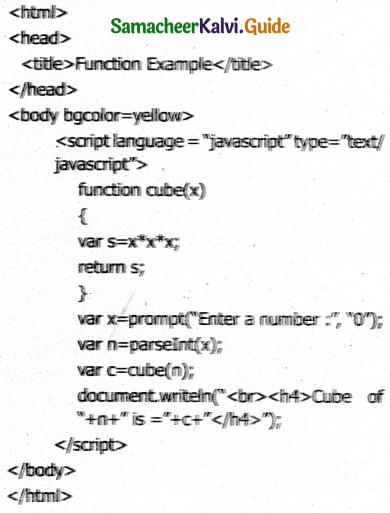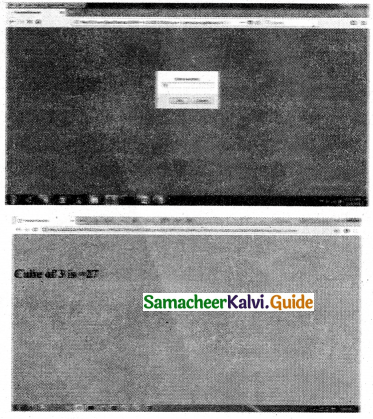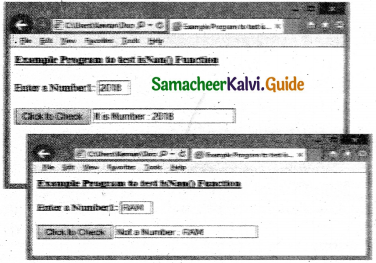Tamilnadu State Board New Syllabus Samacheer Kalvi 11th Computer Applications Guide Pdf Chapter 16 Javascript Functions Text Book Back Questions and Answers, Notes.

## Tamilnadu Samacheer Kalvi 11th Computer Applications Solutions Chapter 16 Javascript Functions

### 11th Computer Applications Guide Javascript Functions Text Book Questions and Answers

Part – I

Question 1.
The parameters work as _______.
a) Local variable
b) Global Variable
c) File variable
d) block variable
a) Local variable

Question 2.
Predefined functions are also called as _______.
a) Library functions
b) storage functions
c) instructions
d) commands
a) Library functions

Question 3.
Larger programs are divided into smaller are called _______.
a) modules
b) block
c) sets
d) group
a) modules

Question 4.
Which of the following is used to enhance reusability and program clarity?
a) functions
b) modules
c) sets
d) instructions
a) functionsQuestion 5.
Which of the following allow the programmer to modularize a program?
a) Library functions
b) User defined functions
c) Normal functions
d) Ordinary functions
b) User defined functions

Part – II

Question 1.
What is a function in JavaScript?
A function is a block of JavaScript code that is defined once but may be executed or invoked any number of times.

Question 2.
What is the use of function?

1. Functions are used to encapsulate code that performs a specific task.
2. Functions are used to keep code that performs a particular job in one place in order to enhance reusability and program clarity.

Question 3.
Write a note on Library functions.
Pre-defined functions are already defined in the JavaScript library which are also called Library functions. For example isNaN(), toUpperCase(), toLowerCase(), length(), alert(), prompt(),write() etc., are pre-defined functions.

Question 4.
Write a note on user defined functions.
User defined functions:

1. User-defined functions allow the programmer to modularize a program.
2. Most computer programs that solve real- world problems are much large, occupy more space in the computer memory and takes more time to execute.
3. Large programs are divided into small programs are called modules.

Question 5.
Write the syntax of functions.
The syntax of a function definition is:
Function function-name(parameters list)Part – III

Question 1.
Write a program in JavaScript to find the cube of a number using function HTML CODE:OUTPUT:Question 2.
Write a program in JavaScript to find the sum of 10 numbers using function.OUTPUT:### 11th Computer Applications Guide Javascript Functions Additional Important Questions and Answers

Part – I

Question 1.
A _______ is a block of JavaScript code that is defined once but may be executed or invoked any number of times.
a) Loop
b) Function
c) Control structures
d) None of these
b) Function

Question 2.
_______ are used to encapsulate code that performs a specific task.
a) Loop
b) Function
c) Control structures
d) None of these
b) Function

Question 3.
_______ are defined for commonly required tasks to avoid the repetition entailed in typing the same statements over and over.
a) Loop
b) Function
c) Control structures
d) None of these
b) FunctionQuestion 4.
_______ are used to keep code that performs a particular job in one place in order to enhance reusability and program clarity.
a) Function
b) Loop
c) Control structures
d) None of these
a) Function

Question 5.
JavaScript functions are _______.
a) Parameterized
b) Non-parameterized
c) Either A or B
d) None of these
c) Either A or B

Question 6.
A _______ function definition may include a list of identifiers.
a) Parameterized
b) Non-parameterized
c) Either A or B
d) None of these
a) Parameterized

Question 7.
A _______ function definition may Include a list of identifiers that work as local variables for the body of the function.
a) Parameterized
b) Non-parameterized
c) Either A or B
d) None of these
a) Parameterized

Question 8.
Identify the correct statement from the following with respect to function.
a) Function invocations provide values or arguments for the function’s parameters.
b) Functions often use their argument values to compute a return value that becomes the value of the function invocation expression.
c) Sometimes functions are defined for commonly required tasks to avoid the repetition entailed in typing the same statements over and over.
d) All the above
d) All the above

Question 9.
JavaScript supports types functions.
a) two
b) three
c) four
d) five
a) two

Question 10.
JavaScript supports _______ function.
a) Pre-defined
b) Library
c) User-defined
d) All the above
d) All the aboveQuestion 11.
Pre-defined functions are already defined in the JavaScript library which are also called _______ functions.
a) Automatic
b) Library
c) User-defined
d) All the above
b) Library

Question 12.
_______ is library function.
a) isNaN()
b) toUpperCase()
c) toLowerCase()
d) All the above
d) All the above

Question 13.
_______ is library function.
b) prompt()
c) write()
d) All the above
d) All the above

Question 14.
_______ is a pre-defined function.
a) toUpperCase()
b) toLowerCase()
d) All the above
d) All the above

Question 15.
_______ is a pre-defined function.
a) parselnt()
b) parseFloat()
c) length()
d) All the above
d) All the above

Question 16.
_______ functions allow the programmer to modularize a program.
a) Pre-defined
b) Library
c) User-defined
d) All the above
c) User-defined

Question 17.
_______ function is used to convert given string into uppercase.
a) toUpperCase()
b) toLowerCase()
d) All the above
a) toUpperCase()

Question 18.
_______ function is used to convert given string into lowercase.
a) toUpperCase()
b) toLowerCase()
d) All the above
b) toLowerCase()

Question 19.
_______ function is used to find the length of the given string.
a) parselnt()
b) parseFloat()
c) length()
d) All the above
c) length()Question 20.
_______ function is used to convert the given value into an integer.
a) parselnt()
b) parseFloat()
c) length()
d) All the above
a) parselnt()

Question 21.
_______ function is used to convert the given string value into an float.
a) parselnt()
b) parseFioat()
c) length()
d) All the above
b) parseFioat()

Question 22.
The large programs are divided into small programs are called _______
a) Modules
b) Blocks
c) Groups
d) None of these
a) Modules

Question 23.
The function-name is any valid _______.
a) Constant
b) Literals
c) Identifier
d) None of these
c) Identifier

Question 24.
Parameter list contains more than one variable then _______ must be there between the variable.
a) Semicolon
b) Comma
c) Colon
d) None of these
b) Comma

Question 25.
The function body must be enclosed by _______.
a) Square brackets
b) Curly braces
c) Angle brackets
d) None of these
c) Angle brackets

Question 26.
The _______ function is used to check whether the given value or variable is valid number.
a) isNaN()
b) toUpperCase()
c) toLowerCase()
d) All the above
a) isNaN()

Question 27.
_______ function returns true if the given value is not a number.
a) isNaN()
b) toUpperCase()
c) toLowerCase()
d) All the above
a) isNaN()

Question 28.
The _______ method returns the element that has the ID attribute with the specified value.
a) getByld()
b) getElementByld()
c) getElementld()
d) None of these
b) getElementByld()

Question 29.
_______ indicates the first option given in the question.
a) elements
b) elements[l]
c) Either A or B
d) None of these
a) elementsPart – II

Question 1.
What do you mean by parameterized function?
A parameterized function definition may include a list of identifiers, known as parameters that work as local variables for the body of the function. Function invocations provide values or arguments for the function’s parameters

Question 2.
What are the functions supported by JavaScript?
JavaScript supports two types functions. They are

1. Pre-defined or Library Functions
2. User-defined Functions

Question 3.
What is pre-defined function?
Pre-defined functions: Pre-defined functions are already defined in the JavaScript library which are also called Library functions.

Question 4.
List some pre-defined functions.
The following are pre-defined functions in JavaScript.

1. isNaN()
2. toUpperCase()
3. toLowerCase()
4. length()
6. prompt()
7. write()Part – III

Question 1.
Tabulate some pre-defined functions and its function with an example.Part – IV

IV. Explain In Brief

Question 1.
Write a program in JavaScript for adding two numbers using function.
HTML CODEOUTPUT:Question 2.
Write a program in JavaScript to test isNan() Function.